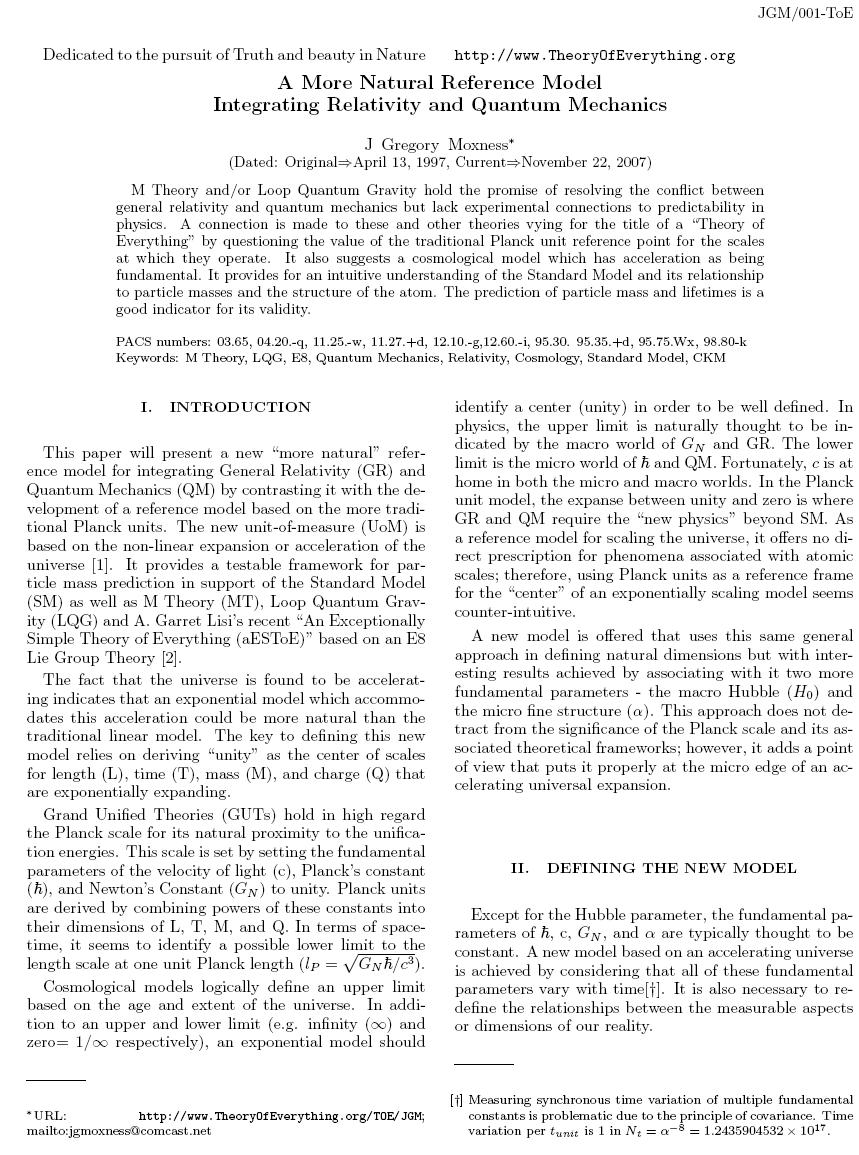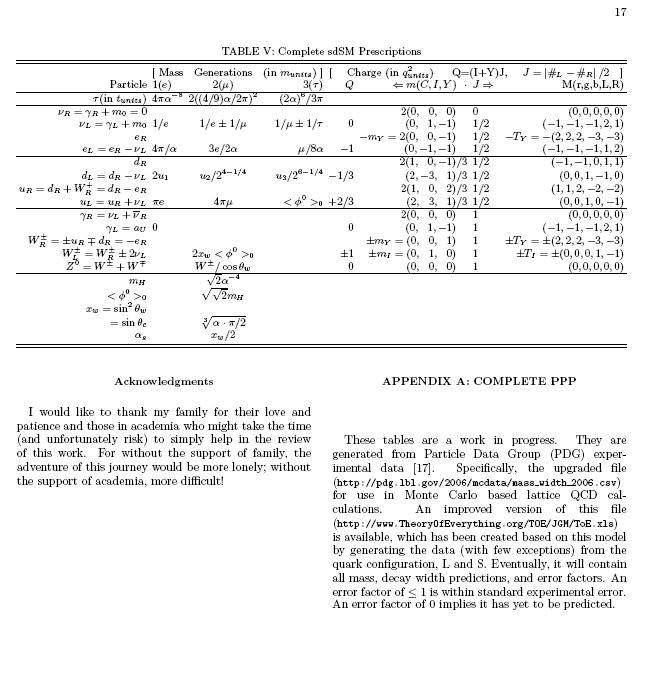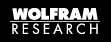## J. Gregory Moxness' Possible Foundation for a TOE Model

### My View

 ToE.pdf My latest paper (full detail of all latest work ToE.ps - would like to get academia peer review) LaTeX APS (RevTex4) publishing format e8Viewer.nbpe8Viewer.nbp - e8 particle viewer This is based on A Garrett Lisi's e8 AESToE model plus my ToE mass/lifetime formula calculations. This is a Mathematica Notebook Player file and can be played on the free Mathematica player from www.wolfram.com. e8Flyer.nbpe8Flyer.nbp - same as the viewer but with added controls to manipulate a flight around the 8D particle configuration space projected onto 2D or 3D space. E8MetaFavorites HTML .ppt screen shots of e8Viewer MetaFavorites E8Favorites HTML .ppt screen shots of e8Viewer Favorites E8MiscFavorites HTML .ppt screen shots of e8Viewer MiscFavorites E8Favorites.pdf A .pdf w/screen shots of e8Viewer MetaFavorites ToEnb.pdf The same paper above validated w/Mathematica 6 What time is it.pdf A TheoryOfEverything in one .ppt slide My Tools of the Trade I modified FormCalc to work on WinTel platforms

A few pages from my paper....This theory is based on (multiple, varying) fundamental Universal Constants linked to Time. It begins with the current and most precise experimental data, the Standard Model (SM), and General Relativity's (GR) expanding (accelerating) Space-Time.

The standard GR definition of time in the context of physics is “the distance between events”. As such, it equates t=l or more properly t=l/c where c=DimensionessUnity for so called ‘Natural Units’.

An extension of this idea is offered based on the fact that the universe is found to be not only following a Hubble expansion, it has been found to be accelerating.

This provides an opportunity to propose a “more natural” Units of Measure (UoM) by extending Planck Units that accounts for this acceleration by normalizing the fundamental universal constants to that acceleration. Consider this normalization to be a kind of “Meta-Relativity”. It associates the fundamental universal constants to time.

The dimensionality associated with space, time, mass and charge are slightly different from those of Planck Units (where c=hBar=G=1):

l=t -> l=t2

m=1/l -> m=l3/t = t5

e=sqrt(hBar*c) = sqrt(mvr*v) = dimensionless -> e= m/sqrt(l) = m/t = l3/t2 = l2 = t4

This clearly implies an acceleration due to an ever increasing velocity of light. The previously presumed “short inflationary period” soon after the big bang is now shown to be a natural and ongoing part of the process of the normal accelerating expansion of the universe.

The obvious question of the source of this acceleration can be addressed by the fact that acceleration is dimensionless (l=t^2, so l/t^2 is dimensionless-- just as in 'Natural Units' l=t, so v is dimensionless). In the context of Meta-Relativity, this can be viewed as the “Meta-Inertial Reference Frame of Meta-Relativity". The source of the acceleration is the creation of particles of matter in quantum fluctuations and the Big Bang.

A natural conjecture for a prescription of the mass of the Higgs boson (148 GeV) is given based on using Higgs mass to define the charge of the electron (e). It is also interesting that with a natural explanation for Dark Energy=2/3, a precise calculation for the age of the universe based on the measured value of fine structure is found to be 13,857,928,235.3 +/-9.7 years.

Related Mathematica include files (typically found in Mathematica_Install_Directory\AddOns\StandardPackages\Miscellaneous\:

 NewUnits.m Units.m with added conversions to the new 'More Natural' Units using a call to UNITS[] NewPhysicalConstants.m PhysicalConstants.m organized for more derived vs. measured fundamental values PhysicalConstantErrors.m Added a file to define physical constant errors organized for derived vs. measured error values

The Compton Effect in Std Theory & Current Time in my theoryThe Compton Effect in my theory at Universe(t=0)Download the Adobe ReaderEvaluate WinZipBuy Mathematica altarelli parisi, big bang, black hole physics, bottom quark, charm quark, charmed quark, critical density, down quark, electromagnetic force, fermi constant, feynman loop, field theory, fine structure, free space impedance, fundamental constant, gamma ray, gauge theory, grand unification, grand unified theory, hubble constant, hubble's constant, inflation period, M theory, mathematical physics, mu meson, natural units, new physics, newton constant, newton's constant, noether's theorem, nuclear astrophysics, nuclear physics, parity violation, particle accelerator, perturbation theory, planck constant, planck units, Planck's constant, quantum cosmology, quantum physics, quantum spin, quark gluon, splitting function, space time, speed of light, standard model, strange quark, string theory, strong force, structure function, super symmetric, super symmetry, theoretical physics, theory of everything, top quark, unit of measure, units of measure, universal constant, up quark, vacuum expectation, velocity of light, weak boson, weak force, weak mixing, black hole singularity, zero point field, arXiv, astrophysics, boson, cern, cosmological, cosmology, CPT, CPT violation, dglap, electroweak, entropic, entropy, fermion, feyncalc, fnal, formcalc, geometrodynamics, gluon, gravitation, gravitational, gravity, higgs, lanl, lbnl, LEP, lepton, looptools, meson, meson particle, muon, neutrino, ornl, particle mass prediction, particle mass prescription, permeability, permitivity, permittivity, phonon, photon, pion, qcd, qed, quark, relativity, singularity, slac, susy, topological, universal acceleration, universe acceleration, ZPF